Wednesday 21st October 2020CBSE Guess > Papers > Important Questions > Class XII > 2009 > Maths > Mathematics By Anil Kumar Tondak

CBSE CLASS XII

Differential Equations

Q. 1. sec2x. tany dx + sec2y. tanx dy = 0.

Q. 2. xdy – ydx =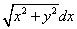Q. 3. 2xy + y2 – 2x2 dy/dx = 0 y = 2 when x = 1

Q. 4. x logx. dy/dx + y = (2/x) logx

Q. 5. y dx + (x – y2) dy = 0

Q. 6. (tan-1y -  x)dy = (1 + y2) dx
Q. 7. (1 + x2)dy/dx + 2xy =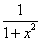y = 0 when x = 1

Q. 8. x2dy + (xy + y2)dx = 0 y = 1 when x = 1

Q. 9. dx/dy + ycotx = 2x + x2cotx y = 0 when x =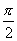Q. 10. (x + y)dy = dx

Q. 11. x (xdy – ydx) = ydx y(1) = 1.

Q. 12. dy/dx = cos(x + y) + sin(x + y)

Q. 13. x dy/dx = y – x tan(y/x).
Q. 14. (x2 + 1) dy/dx + 2xy =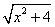Q. 15. (x2 + y2)dx + xy.dy = 0 y(1) = 1

Q. 16. (x + y + 1)2 dy = dx, y(-1) = 0

Q. 17. (xy2 + 2x)dx + (x2y + 2y)dy = 0

Q. 18. dy/dx +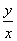= cosx +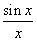Q. 19. Find the differential equation of all circles in the first quadrant which touch the co-ordinate axis.

Q. 20. Form the differential equation corresponding to y2 = m(a2 – x2) by eliminating parameters m and a.

Prepared By Anil Kumar Tondak
M.Sc.(Maths), M.Ed., M.A.(Eco),M.B.A.(Mkt)
Mob No. 9811363962

 Maths